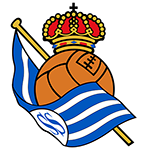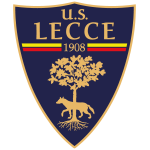# .css-adzqt3{display:inline-block;word-break:break-word;opacity:0;position:relative;-webkit-animation:animation-plwpox 0ms 0.4s forwards;animation:animation-plwpox 0ms 0.4s forwards;}.css-adzqt3::after{content:"";position:absolute;width:calc(100% + 14px);height:100%;top:0px;left:0px;background-color:var(--chakra-colors-light-white_100);-webkit-transform:scale3d(0, 1.05, 1);-moz-transform:scale3d(0, 1.05, 1);-ms-transform:scale3d(0, 1.05, 1);transform:scale3d(0, 1.05, 1);-webkit-animation:animation-f981je 250ms 0.15000000000000002s forwards step-end,animation-1ncn112 250ms 0.65s forwards step-end;animation:animation-f981je 250ms 0.15000000000000002s forwards step-end,animation-1ncn112 250ms 0.65s forwards step-end;}@-webkit-keyframes animation-1ncn112{0%{-webkit-transform:scale3d(0.75, 1.05, 1);-moz-transform:scale3d(0.75, 1.05, 1);-ms-transform:scale3d(0.75, 1.05, 1);transform:scale3d(0.75, 1.05, 1);transform-origin:top right;}44.4%{-webkit-transform:scale3d(0.25, 1.05, 1);-moz-transform:scale3d(0.25, 1.05, 1);-ms-transform:scale3d(0.25, 1.05, 1);transform:scale3d(0.25, 1.05, 1);transform-origin:top right;}100%{-webkit-transform:scale3d(0, 1.05, 1);-moz-transform:scale3d(0, 1.05, 1);-ms-transform:scale3d(0, 1.05, 1);transform:scale3d(0, 1.05, 1);transform-origin:top right;}}@keyframes animation-1ncn112{0%{-webkit-transform:scale3d(0.75, 1.05, 1);-moz-transform:scale3d(0.75, 1.05, 1);-ms-transform:scale3d(0.75, 1.05, 1);transform:scale3d(0.75, 1.05, 1);transform-origin:top right;}44.4%{-webkit-transform:scale3d(0.25, 1.05, 1);-moz-transform:scale3d(0.25, 1.05, 1);-ms-transform:scale3d(0.25, 1.05, 1);transform:scale3d(0.25, 1.05, 1);transform-origin:top right;}100%{-webkit-transform:scale3d(0, 1.05, 1);-moz-transform:scale3d(0, 1.05, 1);-ms-transform:scale3d(0, 1.05, 1);transform:scale3d(0, 1.05, 1);transform-origin:top right;}}@-webkit-keyframes animation-f981je{0%{-webkit-transform:scale3d(0, 1.05, 1);-moz-transform:scale3d(0, 1.05, 1);-ms-transform:scale3d(0, 1.05, 1);transform:scale3d(0, 1.05, 1);transform-origin:top left;}22.2%{-webkit-transform:scale3d(0.25, 1.05, 1);-moz-transform:scale3d(0.25, 1.05, 1);-ms-transform:scale3d(0.25, 1.05, 1);transform:scale3d(0.25, 1.05, 1);transform-origin:top left;}44.4%{-webkit-transform:scale3d(0.5, 1.05, 1);-moz-transform:scale3d(0.5, 1.05, 1);-ms-transform:scale3d(0.5, 1.05, 1);transform:scale3d(0.5, 1.05, 1);transform-origin:top left;}66.6%{-webkit-transform:scale3d(0.75, 1.05, 1);-moz-transform:scale3d(0.75, 1.05, 1);-ms-transform:scale3d(0.75, 1.05, 1);transform:scale3d(0.75, 1.05, 1);transform-origin:top left;}100%{-webkit-transform:scale3d(1, 1.05, 1);-moz-transform:scale3d(1, 1.05, 1);-ms-transform:scale3d(1, 1.05, 1);transform:scale3d(1, 1.05, 1);transform-origin:top left;}}@keyframes animation-f981je{0%{-webkit-transform:scale3d(0, 1.05, 1);-moz-transform:scale3d(0, 1.05, 1);-ms-transform:scale3d(0, 1.05, 1);transform:scale3d(0, 1.05, 1);transform-origin:top left;}22.2%{-webkit-transform:scale3d(0.25, 1.05, 1);-moz-transform:scale3d(0.25, 1.05, 1);-ms-transform:scale3d(0.25, 1.05, 1);transform:scale3d(0.25, 1.05, 1);transform-origin:top left;}44.4%{-webkit-transform:scale3d(0.5, 1.05, 1);-moz-transform:scale3d(0.5, 1.05, 1);-ms-transform:scale3d(0.5, 1.05, 1);transform:scale3d(0.5, 1.05, 1);transform-origin:top left;}66.6%{-webkit-transform:scale3d(0.75, 1.05, 1);-moz-transform:scale3d(0.75, 1.05, 1);-ms-transform:scale3d(0.75, 1.05, 1);transform:scale3d(0.75, 1.05, 1);transform-origin:top left;}100%{-webkit-transform:scale3d(1, 1.05, 1);-moz-transform:scale3d(1, 1.05, 1);-ms-transform:scale3d(1, 1.05, 1);transform:scale3d(1, 1.05, 1);transform-origin:top left;}}@-webkit-keyframes animation-plwpox{from{opacity:0;}to{opacity:1;}}@keyframes animation-plwpox{from{opacity:0;}to{opacity:1;}}SEASON• December 2023
• Champions League国米

Dec 12th 2023 — 20:00皇家社会
• Serie A TIM拉齐奥

Dec 17th 2023 — 19:45国米
• Coppa Italia Frecciarossa国米

Dec 20th 2023 — 20:00博洛尼亚
• Serie A TIM国米

Dec 23rd 2023 — 17:00莱切
•热那亚

Dec 29th 2023 — 19:45国米
• January 2024
• Serie A TIM国米

Jan 6th 2024 — 11:30维罗纳
•蒙扎

Jan 14th 2024 — 14:00

Date not confirmed国米

## 意甲standings

G

W

D

L

GS

P

115

12

2

1

37-7

38

215

11

3

1

23-9

36

315

9

2

4

26-18

29

415

7

4

4

28-17

25

• 俱乐部

• Inter Store